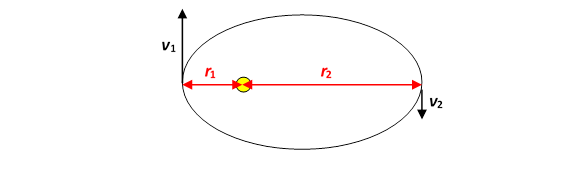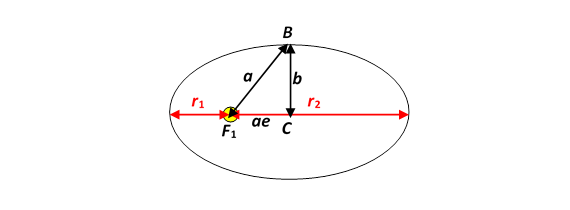# Elliptic Orbits: Paths to the Planets

Michael Fowler

Try aiming for Mars yourself with this applet.

### Deriving Essential Properties of Elliptic Orbits

From a practical point of view, elliptical orbits are a lot more important than circular orbits.  A spaceship leaving earth and going in a circular orbit won’t get very far. And although proving the planetary orbits are elliptical is quite a tricky exercise (the details can be found in the last section of the Discovering Gravity lecture), once that is established a lot can be deduced without further fancy mathematics.

Think about an astronaut planning a voyage from earth to Mars. The two important questions (apart from can I get back?) are:

How much fuel will this trip need?

How much time will it take?

It is crucial to minimize the fuel requirement, because lifting fuel into orbit is extremely expensive.

Ignoring minor refinements like midcourse corrections, the spaceship’s trajectory to Mars will be along an elliptical path.  We can calculate the amount of fuel required if we know the total energy of the ship in this elliptical path, and we can calculate the time needed if we know the orbital time in the elliptical path because, as will become apparent, following the most fuel-efficient path will take the ship exactly half way round the ellipse.

Remarkably, for a spaceship (or a planet) in an elliptical orbit, both the total energy and the orbital time depend only on the length of the major axis of the ellipse$—$as we shall soon show.

Visualizing the orbit of the spaceship going to Mars, and remembering it is an ellipse with the Sun at one focus, the smallest ellipse we can manage has the point furthest from the Sun at Mars, and the point nearest to the Sun at earth.

(Important Exercise:  Sketch the orbits of earth and Mars, and this elliptical trajectory--then check your sketch with the applet!)

This then immediately gives us the major axis of this smallest ellipse, so we can figure out, from the results given below, how much fuel and time this will take.

Here are the two basic relevant facts about elliptical orbits:

1.  The time to go around an elliptical orbit once depends only on the length a of the semimajor axis, not on the length of the minor axis:

$T 2 = 4 π 2 a 3 GM .$

2.  The total energy of a planet in an elliptical orbit depends only on the length a of the semimajor axis, not on the length of the minor axis:

$E tot =− GMm 2a .$

These results will get you a long way in understanding the orbits of planets, asteroids, spaceships and so on$—$and, given that the orbits are elliptical, they are fairly easy to prove.

They follow from the two conservation laws:

1.  Total energy stays constant,

2.  Angular momentum stays constant,

throughout the elliptical orbital motion.

We’ll derive the results for a planet, beginning with the conservation laws.  In fact, it turns out that all we need to use is that the energy and angular momentum are the same at the two extreme points of the orbit:Labeling the distance of closest approach $r 1 ,$ and the speed at that point $v 1 ,$ the furthest point $r 2 ,$ the speed there $v 2 ,$ we have

$m v 1 r 1 =m v 2 r 2 =L$

and

$1 2 m v 1 2 −GMm/ r 1 = 1 2 m v 2 2 −GMm/ r 2 =E.$

From the second equation,

$1 2 m v 1 2 −GMm/ r 1 −( 1 2 m v 2 2 −GMm/ r 2 )=0.$

Rearranging, and dropping the common factor $m,$

$1 2 ( v 1 2 − v 2 2 )=GM( 1 r 1 − 1 r 2 ).$

Using the angular momentum equation to write $v 1 =L/m r 1 , v 2 =L/m r 2 ,$ and substituting these values in this equation gives $L 2$ in terms of $r 1 , r 2 :$

$L 2 2 m 2 ( 1 r 1 2 − 1 r 2 2 )= L 2 2 m 2 ( 1 r 1 + 1 r 2 )( 1 r 1 − 1 r 2 )=GM( 1 r 1 − 1 r 2 )$

from which

$L 2 2 m 2 = GM ( 1 r 1 + 1 r 2 ) .$

The area of the ellipse is $πab$ (recall it’s a circle squashed by a factor $b/a$ in one direction, so $π a 2$ becomes $πab$ ), and the rate of sweeping out of area is $L/2m,$ so the time $T$ for a complete orbit is given by:

$T 2 = ( πab ) 2 L 2 /4 m 2 = 2 ( πab ) 2 GM ( 1 r 1 + 1 r 2 ).$

To make further progress in proving the orbital time $T$ depends on $a$ but not on $b,$ we need to express $r 1 , r 2$ in terms of $a$ and $b.$

### Useful Ellipse Factoid

Recall that the Sun is at a focus $F 1$ of the elliptical path (see figure below), and (from the “string” definition of the ellipse) the distance from the Sun to point $B$ at the end of the minor axis is $a.$  Pythagoras’ theorem applied to the triangle $F 1 BC$ gives

$a 2 ( 1− e 2 )= b 2$

and from the figure

$r 1 =a( 1−e ) r 2 =a( 1+e )$Therefore

$r 1 r 2 = b 2 .$

Also from the figure

$r 1 + r 2 2 =a,$

so we have the amusing result that

the semimajor axis $a$ is the arithmetic mean of $r 1 , r 2$  and the semi-minor axis $b$ is their geometric mean,

and furthermore

$( 1 r 1 + 1 r 2 )= r 1 + r 2 r 1 r 2 = 2a b 2 .$

### Deriving Kepler’s Law

We can immediately use the above result to express the angular momentum $L$ very simply:

$L 2 2 m 2 = GM ( 1 r 1 + 1 r 2 ) = GM b 2 2a .$

We’re now ready to find the time for one orbit $T.$   Remember $T$ is the total area of the orbit divided by the rate area is swept out, and that rate is $L/2m,$  so:

$T 2 = ( πab ) 2 L 2 /4 m 2 = 2 ( πab ) 2 2a GM b 2 = 4 π 2 a 3 GM .$

That is,

$T 2 = 4 π 2 a 3 GM ,$

a simple generalization of the result for circular orbits.

To prove that the total energy only depends on the length of the major axis, we simply add the total energies at the two extreme points:

$1 2 m v 1 2 −GMm/ r 1 + 1 2 m v 2 2 −GMm/ r 2 =2E.$

The substitution $v 1 =L/m r 1 , v 2 =L/m r 2$ in this equation gives

$L 2 2m ( 1 r 1 2 + 1 r 2 2 )−GMm( 1 r 1 + 1 r 2 )=2E.$

Writing

$( 1 r 1 2 + 1 r 2 2 )= ( 1 r 1 + 1 r 2 ) 2 − 2 r 1 r 2 ,$

it is easy to check that

$E=− GM r 1 + r 2 =− GM 2a$

where a is the semimajor axis.

Exercise:  From $L 2 2 m 2 = GM b 2 2a ,$ find the speed of the planet at it goes through the point $B$ at the end of the minor axis.  What is its potential energy at that point?  Deduce that the total energy depends only on the length of the major axis. (This is an alternative derivation.)

### Hyperbolic (and Parabolic?) Orbits

Imagining the satellite as a particle sliding around in a frictionless well representing the potential energy as pictured above, one can see how both circular and elliptical orbits might occur.

(Optional: More formally, we solved the equation of motion at the end of these earlier notes to find

$1 r = GM m 2 L 2 +Acosθ$

which is equivalent to the equation for an ellipse

$a( 1− e 2 ) r =1+ecosθ$

as discussed there.)

However, that is not the whole story:  what if a rogue planet comes flying towards the Solar System from outer space?  What kind of orbit will it follow as it encounters the Sun’s gravity?  In fact, our analysis of the equations of motion is equally valid in this case, and the $( r,θ )$ equation is the same as that above!  The new wrinkle is that $e,$ which is always less than one for an ellipse, becomes greater than one, and this means that for some angles $r$ can be infinite (the right-hand side of the above equation can be zero). The orbit is a hyperbola: the rogue comes in almost along a straight line at large distances, the Sun’s gravity causes it to deviate, it swings around the Sun, then recedes tending to another straight line path as it leaves the System.

There is also the theoretical possibility of a parabolic orbit, going out to infinity but never approaching a straight line asymptote.  However, this requires exactly the correct energy$—$the slightest difference would turn it into a very long ellipse or a hyperbola.  In practice, of course, this delicate energy tuning would be upset by gravitational attraction from other planets.

### Really Getting Out There: the Slingshot

Although the elliptic orbit touching the (approximately) circular orbits of earth and Mars is the most economical orbit of getting to Mars, trips to the outer planets can get help.  Imagine a slowly moving spaceship reaching Jupiter’s orbit at a point some distance in front of Jupiter as Jupiter moves along the orbit.  In Jupiter’s frame of reference, this ship is moving towards Jupiter at a speed roughly equal to Jupiter’s own speed relative to the Sun.  In Jupiter’s frame, assuming the spaceship is sufficiently far from the orbit that it doesn’t crash into Jupiter, it will fall towards Jupiter, swing around the back, and then be flung forward. In the Sun’s frame, the gravitational pull on the spaceship from Jupiter was strongest as the spaceship swung behind Jupiter, and this pull accelerated the spaceship in the same direction Jupiter moves in the orbit, so the spaceship subsequently moves ahead of Jupiter, having gained enough energy to move further out in the solar system.  This is at the expense of Jupiter: during the time the spaceship was swinging behind Jupiter, it slowed Jupiter’s orbital speed$—$but not much!   The slingshot is obviously a delicate operation: you don’t want to crash into Jupiter, but also you don’t want to be trapped in an elliptic orbit around Jupiter.  But the benefits are so great that in practice all spaceships venturing to the outer planets use it, often more than once.